Natural Length Contraction Mechanism Due to Kinetic Energy

Paul Marmet

( Last checked 2018/01/15 - The estate of Paul Marmet )

Eine deutsche Übersetzung dieses Artikels finden Sie hier.

Abstract.
This paper shows that the phenomena usually attributed to relativity are a simple consequence of mass-energy conservation.  When atoms are accelerated, the increase of kinetic energy increases the electron mass, which makes the Bohr radius larger.  This increase of radius produces a shift in the atomic energy levels and also an increase of the physical size of matter.  Consequently, a moving atomic clock now runs at a different rate.  Quite naturally and without Einstein's relativity, we see how the increase of size of the Bohr radius and of macroscopic matter, are exactly equal to Einstein's prediction.  Einstein's theory of relativity predicts length contraction, but does not explain how matter can be physically contracted or why this phenomenon is not reversible when the mass in the moving frame is accelerated back to the original frame.  Einstein's length contraction implies that the Bohr atom gets smaller.  However, quantum mechanics shows that such a contraction of the Bohr radius should increase the atomic energy levels.  This consequence of Einstein's predictions is contrary to observational facts, which show that, at high velocity, the atomic energy levels become smaller and the atomic clocks get slower.  The mechanism of dilation and contraction of matter is logically explained here, using Newton physics and the fundamental principles of quantum mechanics.  Using the de Broglie equation, we calculate the relationship between the Bohr radii in different frames, which is responsible for the physical change of length of matter, in agreement with all observational data.  Furthermore, just as for mass and energy units, we show that the physical size of the Planck unit, needs to increase g times with velocity. Observations previously attributed to relativity can now be explained logically.  These results are also compatible with a rational explanation of the advance of the perihelion of Mercury around the Sun.  We must conclude that there exists no space-time distortion and the Einstein's relativity principle cannot be valid.  Everything is naturally explained as a change of size of matter and a change of clock rate.  A corresponding solution also exists in the case of gravitational energy as will be demonstrated in a future paper.  These phenomena, taking place in atoms are also predictable in the nucleus of matter.  For the same reason, the lifetimes of radioactive nuclear states also changes naturally with velocity and potential energy.

1- Introduction.
The problem of dilation and contraction of matter in relativity could never be explained logically.  Einstein's relativity presents no physical rationalization explaining why and how matter can dilate or contract.  That field of physics is impenetrable, because it is not compatible with the existence of a physical reality, independent of the observer's existence.  Einstein's theory has never been expressed unequivocally and the more recent theoretical developments collapse into a deeper mystery.  Unfortunately, just as during the Middle Ages, most scientists accept the idea that nature is not compatible with conventional logic.  Nowadays, most scientists ignore or refuse to read papers implying an existence of matter independent of the observer.
In this paper, the phenomenon of length contraction or dilation is explained logically without any of Einstein's relativity hypotheses (1).  Everything is explained as a function of the physics of Newton, Coulomb and de Broglie.  Due to the increase of kinetic energy and the application of the principle of mass-energy conservation, we see that the Bohr radius increases, so that the physical size of matter increases.  This dilation of matter is not a simple mathematical transformation visible by only one observer in a specific frame, it is a physical reality.  Also, matter shrinks back to its original length when the velocity is reduced.  The fundamental principles related to this natural phenomenon have been explained previously (2)
The fundamental reason for which the Bohr radius increases when kinetic energy is given to the atom is mechanical.  In a few words, atoms in space are like gyroscopes moving freely in space.  The orbiting electron around the nucleus represents the spinning wheel.  When the mass of the spinning wheel (here the orbiting electron inside an atom) increases, the velocity of rotation decreases, due to momentum conservation.  Therefore in the Bohr atom, when the electron mass increases, the electron velocity decreases.  Using Coulomb forces, it is well known that the radius of the electron orbit is larger when the electron velocity becomes slower.  Consequently, the Bohr radius becomes larger when the atom is moving faster.  The change of Bohr radius is the fundamental cause of dilation of matter when atoms acquire kinetic energy.  This mechanism is calculated in detail in this paper.
In agreement with observations, we show also that, even if in fact the physical size of the atom is increasing, the quantum structure of the moving atom is changing in such a way that these changes of mass and length remain generally undetectable to the moving observer.  The esoteric Einstein's hypothesis of space-time distortion is unacceptable, because it is not compatible with a physical reality independent of the observer.  Contrary to most papers in modern physics, we always refer here to a realistic physical model.  We question the physical interpretation of equations.  There must be no mathematical model in physics, without being logically supported by a physical model.  One of the most important errors in Einstein's relativity is that, the inevitable change of sizes of some moving reference units (i.e. the Planck constant) are disregarded.
We show here that all phenomena previously attributed to Einstein's relativity are in fact, the simple consequence of application of the principle of mass-energy conservation in atoms and molecules.  There is no time dilation or space contraction.  There is only a change of clock rate and a change of length of matter due to the change of Bohr radius.

2 -Fundamental Mechanisms Inside Atoms.
The complex internal structure of atoms and molecules is nothing but the sum of a few simple fundamental relationships.  The best way to verify the compatibility between all these fundamental relationships and experimental data is with simple atoms, in which each individual phenomenon is recognizable independently.  For example, it is well known that all the deepest fundamental physics phenomena involved in the structure of complex matter, appear under their simplest fundamental form in atomic hydrogen.  Inside the hydrogen atom, we know that the Coulomb attracting force between the two charged particles (electron and the proton) is equal to the Newton centrifugal force of the orbiting electron.  This was recognized by Bohr.  We have: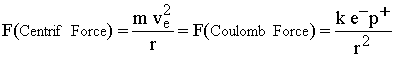1
Where k is the Coulomb constant, e- is the electron charge, p+  is the proton charge, m is the electron mass and r is the average radius of the electron orbit, which is the Bohr radius.  The electron is orbiting the nucleus at a velocity ve.  Furthermore, as discovered by de Broglie, the basic principle of quantum mechanics is satisfied when the circumference of the electron orbit is equal to an integer number n of the de Broglie electron wavelength lB .  This relationship is explained by the Nobel Laureate G. Herzberg in his book "Atomic Spectra and Atomic Structure" (3).  In atomic physics, the parameter n is called the principal quantum number and r is the radius of the electron orbit around the nucleus.  This gives: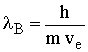and2
The lowest (fundamental) quantum level of the atom is obtained, when the number of de Broglie electron wavelengths n is equal to unity.  A complete Rydberg series of quantum levels is obtained when n equals the integers 1, 2, 3,  . . .   This means that we can have 1, 2, 3 or more integers of the de Broglie electron wavelengths in a complete circumference of the electron orbit.  For any of these quantum numbers, we observe that the de Broglie electron wave is always in phase, after each complete rotation.  All the atomic energy levels of hydrogen observed experimentally correspond to the simultaneous application of relationships 1 and 2.
Equations 1 and 2 are in a perfect agreement with experiments when the atom is stationary.  It is also an experimental fact that when an atom is in motion, the same relationships are compatible within the moving atom, if we use the relevant moving reference units [v].   However, we have seen previously (2) that in fact, the moving atoms are unquestionably different, due to the absorbed kinetic energy, which gives an extra mass to the electron and to the nucleus.  The resulting change of size of the reference units is due to the increase of electron mass.  These previously ignored absolute physical modifications between frames are the origin of the non-realistic relativity principle hypothesized by Einstein.  In order to take into account that both matter and the size of the reference units are changing simultaneously with velocity, we must note that a physical quantity cannot be defined as a simple "number of reference units" as generally used in papers.  In contrast with a mathematical quantity, we must define a physical quantity. A physical quantity is an absolute quantity, defined as the product of the number of units, multiplied by the size of the corresponding reference unit.
Due to the increase of mass with velocity, the size of the reference unit is changing in different frames. This variation of size of reference units must be taken into account.  We have seen previously (2), that we need a double index to get the relevant information on the physical quantity being measured.  For example, we see that the number representing a mass m, can have four different values, depending on the frame where it is located and the reference unit used to measure it.  We can have ms[s], ms[v], mv[s] and mv[v].  The subscript after the physical quantity refers to the frame where the particle is located.  The subscript s means that the particle is located in the stationary frame, and the subscript v means that the particle is located in the moving frame.  Furthermore, the physical quantity is also followed by a square parenthesis, which indicates the size of the fundamental reference unit used to express that physical quantity.  The index [s] means that the corresponding reference unit used is in the stationary frame.  The index [v] means that the reference unit used for that measurement is in the moving frame.
We will see that there exist naturally three different situations when matter moves to a moving frame.  In most cases, an absolute physical quantity like a mass m, is the product of the number of units (ms or mv) times the size of the unit used to measure it, which is [s] or [v].  For example, an absolute physical length like rs[v] is the product of the number of units rs, times the size of the unit [v].   In the second situation, the absolute size of the Coulomb and the Planck constants must be considered when switching frames.  In the Coulomb case, nothing changes neither physically nor mathematically.  This is the case for the electric charges e- and protons p+. In that case, indexes are irrelevant here, since the number of units and the size of units are identical in all frames at any velocity.  It is well known that the absolute electric charge of electrons and protons is constant when the particle is accelerated to high velocity.  This is an experimental fact, since observational data have shown that, when an electron is accelerated to a high velocity and then deflected in a magnetic field, the electron "charge to mass ratio" (e/m) is changing in a way, which is exactly compatible with the expected increase of mass, assuming a constant electric charge and mass-energy conservation.  Consequently, we have:3
The same relationship also exists for the proton.  The absolute electric charge of the positive proton is independent of the velocity.  This gives:4
We have seen previously (2) that the electron mass increases g times when the atom is accelerated to velocity va. This has been demonstrated previously (see Web.) Due to the principle of mass energy conservation, we have seen that the mass of all bodies increases with velocity, following the increase of kinetic energy.  In that case, we have seen previously(2) that the mass of all particles like atoms, protons and electrons increases according to:5
Where:6
In equation 6, v is the absolute velocity of particles (electron, proton or atom), using stationary units.  Equations 5 and 6 only mean that when a particle or even a macroscopic body is accelerated to a moving frame, there is a real physical increase of mass due to the addition of the external kinetic energy to that mass.
In physics, the parameters in equations generally represent the number of standard units, (independently of the size of the unit).  It is arbitrarily assumed that the size of the reference unit is constant in different frames.  However, it is not so when a moving observer is moving with the mass, because the reference units in the moving frame are different, due to their kinetic energy.   Then, the number (alone) of units, to measure masses, lengths and clock rates is insufficient to represent a physical quantity.
An example would be useful.  Let us consider a rod having a length of 2.4 meters when measured in a stationary frame with respect to a reference meter also in the same frame.  This length is written 2.4 ms[s].  If furthermore, that rod is carried to a frame moving at velocity v and is measured with respect to the reference meter also in the local frame (the moving frame), the length of the same rod will be given as 2.4 mv[v].  We see that, in both cases, the length of the rod is mathematically the same (i.e. 2.4 local meters).  However, the physical length of the rod is certainly different in the moving frame.  Also, the physical length given by g times 2.4 ms[s] is equal to 2.4 mv[v].   Furthermore, the physical length 2.4 mv[s] equals 2.4 mv[v].  The reader must cautiously perceive the difference between the mathematical equality and the physical equality.  Realistically, numbers are the only things mathematical equations are calculating.
The usual equations in physics completely rely on an assumption of a definition of a reference unit, which is assumed to be constant in any frame.  This hypothesis is erroneous.  That hypothesis is not compatible with the principle of mass-energy conservation (2)
The parameters in a normal mathematical equation give nothing but the number of units rather than the size of the physical quantity.  In previous papers (2, 4-7), the same number of units was instead represented by the notation N-r, N-m and N-E.

3 - The Coulomb Energy Curve
Atom at Rest. - The equilibrium between the centrifugal force and the electric force between the electron and the nucleus is described in the Bohr model.  This is somewhat similar to the attracting gravitational force between the orbiting planets around the Sun as presented by Gerhard Herzberg (3) and others.  When the atom is stationary, equation 1 gives the relationship between the radius r of the orbiting electron as a function of the orbiting velocity ve.  From equation 1 we get:7
As explained above, the index [s] means that we are using the reference units existing in the stationary frame.  In a stationary frame, the term ke-p+/ms is a physical constant.   Using equation 7 in classical physics, (but without quantum mechanics) the radius of curvature r of the electron orbit is inversely proportional (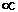) to the square of the velocity.  We have:8
Equation 8 is also compatible with the behavior of the orbit of planets moving around the Sun described by Newton and Kepler.  It is found that the velocity of the planets decreases as the square root of the distance from the Sun.  Similarly, in the case of the electron inside the atom, the electrical potential energy E of the electron corresponding to equation 1 is:9
In equation 9, we see that the radius r of the electron orbit can vary continuously, as long as the quantization of quantum mechanics is ignored.  However, in the microscopic world of quantum mechanics, there is another constraint given by equation 2, which requires that the circumference of the electron orbit be equal to an integer number of de Broglie wavelengths.  This is the fundamental mechanism of quantization.  The Newton and Coulomb equations above must always be satisfied in quantum mechanics, but there is a further essential requirement given by the de Broglie equation, due to the wave nature of matter.   This de Broglie constraint will be calculated below.  Let us simplify the notation using only v instead of ve for the electron velocity.
Atom in Motion.  - When an atom is in motion, due to the kinetic energy transferred from the external frame, (which is added to the particle), the principle of mass-energy conservation requires that the mass of all particles increases.  Therefore, as given by equation 5, the electron mass increases inside the atom, due to the higher velocity of the atom, just as the proton mass.  Let us calculate the change of the electron orbital velocity inside the atom, and around the nucleus, due to the increase of electron mass (from ms[s] to gms[s]).  Substituting gms[s] in equation 7 gives:10
In equation 10, we have assumed momentarily that the Bohr radius remains rs[s].  We will see below that this is not compatible with the de Broglie equation.  We have seen previously (2) that in order to satisfy the principle of mass-energy conservation, the size of the Bohr radius must necessarily increase g times as a function of the velocity of the atom.  This is in perfect agreement with the calculations and observations (2).  This increase of the Bohr radius is tested here.   This absolute increase of size of the Bohr radius, as a function of the velocity of the atom is:11
In that case, let us calculate the electron velocity vv in the moving atom, (when the Bohr radius is grs[s]).  This increase of rs with equation 10 gives:12
In equation 12, the physical transformations related to the motion of the atom are taken into account.  However, we recall that we are still using the [s] reference units.  Let us calculate now the relative electron velocity around the nucleus for the stationary atom (equation 7), with the corresponding electron velocity due to the increase of electron mass and of the Bohr radius.  Equations 7 and 12 give: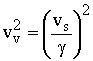13
Equation 13, also gives: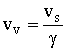14
Equation 14 shows that when the atom is accelerated to velocity v, the electron velocity around the nucleus is reduced g times.  Of course, we have seen previously that the size of the reference unit "velocity" is the same in all frames.  From equations 11 and 14 we have:15
As a consequence of an increase of atom velocity (not the increase of electron velocity inside the atom), equation 15 means that the electron velocity inside the atom varies as the inverse of the radius of the electron orbit.  Using the same notation as in equation 8, equation 15 gives:16
We must notice the difference between equations 16 and 8.  In equation 8, we are changing directly the electron velocity inside an atom.  In equation 16, the change of electron energy is indirect, because it is the consequence of the change of velocity of the atom.  In order to picture more clearly the consequence of the increase of distance between the electron and the nucleus due to the increase of atom velocity, let us represent on figure 1, the Coulomb potential as a function of the distance between the electron and the nucleus.Figure 1
On figure 1, the two heavy curves represent the Coulomb potential given in equation 1.  The orbit (ellipse) As represents the electron orbit of the stationary hydrogen atom in its ground quantum state (ns=1) where n is the principal quantum number.  The radius of this orbit is rs.  Of course, other quantum states like Bs (ns=2) and other states above, exist at higher electron energy in the Coulomb curve in agreement with equation 2 when the atom velocity is zero.  When the atom is accelerated to high velocity, the electron mass becomes larger, so that the series of quantum states As, Bsand the other states above, are shifted to the series of quantum states Av, Bv etc... .  The Bohr radii of all the corresponding quantum states are shifted to a larger radius.  The atom in motion becomes in the quantum states nv=1, 2, 3 etc... as illustrated on figure 1.

4 - Physical Transformations.
When the atom moves from the [s] frame to the [v] frame, we have seen that there is an increase of the Bohr radius, which increases physically from rs[s] to grs[s] (which is physically equal to  rv[v]) .  Due to this increase of radius (equation 11) there is a decrease of energy in the atom that leads to the energy Ev[s] in the moving atom.  Using [s] units, the electrostatic energy between the electron and the proton is: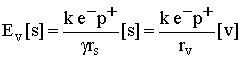17
In equation 17, we see that when the atom is located in the [v] frame and using the [v] reference units, using classical physics, we get the same mathematical relationship as the one using the stationary units, with the atom in the stationary frame.  However, apart from the mathematical relationship, which is the same when the atom is transferred to the moving frame, there is one physical change that took place during these transformations.  When calculating the Bohr radius, the same mathematical relationship does not mean the same absolute energy.  Due to the transfer of the atom to the moving frame, the Bohr radius increases from rs to grs.  The dilated atom is then in the moving frame.  Let us calculate the absolute energy of that atom.  Substituting the Bohr radius rs for grs in equation 9, we see that the absolute energy Ev[s] is g times smaller, when the atom is in the moving frame.  This gives: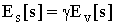18
In order to be able to compare the internal energy inside the atom when it moves from the stationary frame to the moving frame, equation 18 is claculated using the same reference [s] units.  Equation 18 shows that the absolute amount of energy available in quantum transitions is also smaller in the moving frame, although the mathematical relationship is the same.  This is in perfect agreement with all experimental data, since it is also an experimental fact that the quantum transitions emitted by moving atoms possesses a smaller amount of energy.  Therefore the moving observer must utilize the same classical mathematical relationships when he uses the units existing in his moving frame.  We must conclude that the observer in any moving frame will always get the correct answer when using the same mathematical relationship, but the use of the proper units leads to a different absolute energy.
This result is not compatible with the Einstein's invariance principle, because even if the same equations are valid, they do not represent the same absolute amount of energy.   All the quantum transitions appear the same internally, in all frames, because the size of the reference units changes in a way that compensate exactly for the change of size of the physical quantities.  Furthermore, the explanation here is compatible with a physical reality, independent of the observer, contrary to Einstein's relativity.  There is no time dilation, no space contraction.  It is a simple moving atom, which naturally will emit a lower absolute frequency as observed experimentally.

5 - Quantum Conditions - De Broglie Wavelength.
We have seen that inside a stationary atom, as well as inside a moving atom, the Newton's centrifugal force on the orbiting electron is equal and in the opposite direction to the Coulomb force.  We have shown above that this requirement is perfectly satisfied inside the atom when we use the stationary units when the atom is stationary, and when we use the moving units when the atom is moving.  This is done logically without using the Einstein's relativity hypothesis.
However, there is another condition that needs to be verified to be compatible with quantum mechanics.  We have seen in equation 2, that due to quantum mechanics, the atom must be compatible with the de Broglie equation. This condition is fundamental and corresponds to the quantization of electron energy in atoms.  This quantum condition requires that the circumference of the electron orbit is equal to an integer n, times the de Broglie electron wavelength lB.  In atomic physics, this integer is called the principal quantum number.  Let us consider the lowest principal quantum number (when n equals unity).  We can show also that all other quantum numbers (for n=2, 3, 4, etc.) satisfy the solution presented here.  According to de Broglie, the circumference of the lowest electron orbit in an atom in a stationary frame must be:19
Since it is an experimental fact that we can always apply successfully the same equation in all frames, we need to show that equation 19 must also be valid for a moving atom, when we use moving reference units.  We show here that the phenomenon is also compatible with the equation that uses the moving units which gives:20
Since the Planck constant "h" possesses its own units, (it is not a pure number as p), we will see now that the change of mass and length units (required between frames due to mass-energy conservation), is responsible for the change of size of the reference Planck unit in the moving frame.  We use a dimensional analysis to calculate that change of size of units.  Let us formulate a dimension analysis, based on the well-known energy-frequency relationship giving the energy of a photon.21
Where n[s] is the frequency of the photon measured using a clock located in the stationary frame.  In the moving frame, using the [v] units, in order to be coherent, the relationship corresponding to equation 21 must be: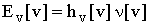22
By definition, the frequency n means the number of cycles per local second, which is defined as the difference of clock display on the local clock.  That local clock is an atomic clock, which counts the number of cycles emitted by a local atom during a time interval.  In physics, a unit of time interval is defined as the time interval during which a standard number of cycles is emitted by an atomic clock.  Consequently, when both an atom to be measured, and also a standard clock are simultaneously moved to a frame at velocity v, the quantum emission rate of the atom to be investigated, as well as the quantum emission rate of the atom which determines the atomic local time, will both vary by the same ratio (g).  Consequently, for any velocity of that frame, the frequency of the atom being studied using the local clock will always be the same.  They both change the same way as given in quantum mechanics.  Consequently, the local frequency is the same in all moving frames when measured with local units. This gives:
ns[s]=nv[v]= n         23
We must also calculate, what is value of the Planck constant h[v] in the moving frame.  In order to calculate the size of the reference Planck constant units existing in different frames, we compare the Planck equations 21 and 22.  Since we consider an absolute amount of energy, the sub index is then useless (always s).   Combining equation 23 with 22 and 21 gives: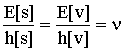24
Also, we have seen in equation 5 that in the moving frame, the size of unit of mass is g times larger than in the size of same mass when it was in the rest frame.  Since there is complete equality between mass and energy, the same relationship holds for energy as for mass.   Therefore a size of the unit of mass is g times larger in the moving frame, just as the size of the unit of energy is g times larger in the moving frame, because they are both related by the constant c2.  Therefore, as seen in equation 5, the relative physical size of the reference units of energy is:25
Equation 25 has nothing in common with equation 18.  We have seen that equation 18 is related to the electron velocity inside the atom.  It gives the change of energy inside the hydrogen atom due to the change of electron velocity, which brings equilibrium between the electronic force and the centrifugal force.  In equation 25, it is the atom velocity, which is involved (not the internal electron).  Equation 25 gives the relative change of local units of energy, due to the important change of velocity of the atom in a moving frame of reference.  Substituting equation 25 in 24 gives: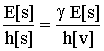26
which gives27
Equation 27 gives the relative size of the reference units of the Planck energy constant between the rest and the moving frame.  Similarly to the increase of energy (or the increase of mass) in the moving frame, equation 27 shows that the size of the Planck constant is g times larger in the moving frame.  Of course, the number of units giving the Planck constant remains the same in all frames.

6 - Testing the de Broglie Equation.
Let us consider the de Broglie relationship.  When we consider an atom at rest, we know that the electron wavelength in the ground state of the atom is equal to the de Broglie electron wavelength.  Let us verify now, that the moving atom calculated above is compatible with de Broglie equation, even when we use the [v] reference units.  Since this is an experimental fact that the same de Broglie equation is valid in all frames, we need to show that equation 19 must also be valid in a moving atom, when we use moving reference units.  In the stationary frame equation 19 is:28
In the moving frame we must have:29
Starting with a normal atom in the stationary [s] frame, we calculate the same atom, substituting the transformations resulting from mass-energy conservation calculated above.  In a first stage, we make the physical transformations expected to follow from the principle of mass-energy conservation as seen by an observer at rest.  In a second step, we will express the same physical quantities using the moving units instead of the stationary units as seen in the moving frame.
Physical Transformations.
With respect to initial conditions, we have the following transformations.  From equation 11, we see that the Bohr radius increases according to:30
Also, due to the velocity, (see equation 5), the mass increases according to:31
Also, due to the increase of atom velocity, we see from equation 14, that the electron velocity inside the atom decreases according to: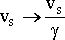32
Local Moving Units.
We have seen in equation 27 that using the same numerical Planck constant, but due to the increase of size of the units in the moving frame, the Planck physical quantity in the moving frame is g times larger (just as for energy in that frame).  For the observer at rest, the larger physical Planck constant (in motion) must be substituted.  Equation 27 gives: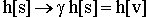33
Substituting equations 30, 31, 32, and 33 in equation 28 gives:34
Equation 34 gives the consequences of mass-energy conservation to atoms.  Let us express the same physical length grs[s] of the Bohr radius but using the longer moving reference units.  This gives:35
Similarly, when the increased mass is measured using the larger reference units in the moving frame, we get:36
Also, we have seen that the electron velocity is g times slower in the moving frame.  In agreement with the description of the moving atom, the electron velocity in the moving frame is g times slower.  This gives:37
Finally, due to the change of units in the moving frame, we have seen in equation 27 that the Planck constant is different in the moving frame according to: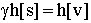38
Substituting equations 35, 36, 37 and 38 in equation 34 give: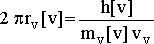39
Equation 39 gives the de Broglie relationship calculated using only the principle of mass-energy, quantum mechanics and classical physics.  We see that the relationship 39 is identical to equation 29.  This shows that the moving observer measuring the structure of his own atoms in his frame will find the same fundamental equations as in quantum mechanics without using any of the Einstein's principle of relativity.  This appears naturally using only conventional logic and mass-energy conservation.

7 - Observations and Discussions.
We must conclude that the moving observer gets the correct physical predictions when he uses the same equation as the stationary observer.  The requirement is that the moving observer must use the moving units and the stationary observer must use the stationary units.  This may appear equivalent to Einstein's principle, which claims that nothing is changed after the acceleration of the frame.  However, Einstein's hypothesis is erroneous, because in fact, the electron velocity, the Bohr radius and the masses of particles are modified.  Einstein did not realize that simple logic and the principle of mass-energy conservation leads to a modification of the reference units in the moving frame that compensates exactly for the real physical changes taking place when masses are accelerated.  Space-time distortions are useless and non-realistic.  Einstein's principle of invariance is an error, because we cannot claim a real invariance in physics when the atoms in different frames have a different Bohr radius, a different electron mass and also emit different frequencies.  As explained in this paper, a numerical invariance is unsatisfactory in physics, because the size of the units inevitably changes between frames.  The physical changes are just perfectly compensated by an equivalent change of the size of the moving reference units of mass, length and clock rate in different frames. Similarly in the Lorentz transformations, nothing takes into account that the size of the units are compelled to change.  Lorentz did not realize that a constant number of units between all frames could simply be explained by the change of size of these units following the principle of mass-energy conservation.  Nature has made the laws of physics so that they appear undistinguishable internally, but this difference can be measured from an external location.
The above results also imply that there is an absolute frame of reference for light so that the velocity of light is (c+v) and (c-v) as confirmed experimentally using the GPS(4).  The GPS system (just as the Sagnac effect) provides a striking proof of an absolute frame of reference for light propagation, but conservatism and non-realism prevent scientists from accepting that evidence.  We must also realize that the change of clock rate and the increase of length of matter is an observational fact.  We can see that the slowing down of moving atomic clocks with velocity requires necessarily an increase of the Bohr radius and therefore an increase of size of matter as shown here.   When we apply Newton mechanics with these classical transformations of length and clock rate to calculate the advance of the perihelion of Mercury, we have shown(5) that they lead naturally to the observed advance, without having to assume the magic of relativity.  This result agrees even with the assumed deflection of light by the Sun(6).   The present analysis also implies that a positive result is expected from the kind of measurements known as the Michelson-Morley experiment.  A recent thorough study(7) of these data has shown that it is so.   Munera's analysis(7) shows that an unbiased analysis reveals different kind of errors, which reveal that there is indeed a shift in optical fringes in the Michelson-Morley type of experiment that has been overlooked previously.
This velocity dependent variation of the internal electronic structure of atoms, does not only exist in the electron shells, but also exists in the nuclear structure.  Since the Planck constant and masses and other fundamental constants are also involved in the nucleus of matter, we can calculate now, the change in the nuclear structure and nuclear forces as a function of the velocity of those nuclei.  Therefore, the change of lifetimes of radioactive nuclei is predictable without Einstein's relativity principles, using the same principle of mass-energy conservation as above.  In the same context, we see that this paper agree with Terrel(8) who found that length contractions and dilations are not measurable to the observer in the moving frame.  This work has also some similarities with the work of Ives(9)  who also used energy and momentum conservation.  Also Munera(10) found an increase of mass in a gravitational and in an electric field.
Finally, it is important to realize that a similar modification of the structure of atoms can be calculated when the energy of the electron inside the atom is perturbed due to gravitational potential, instead of kinetic energy as calculated here.  There has been a calculation of that effect previously(2), but the complete detailed explanation will be published in a coming paper.  We will see that the change of gravitational energy can explain logically all the phenomena previously requiring the Einstein's hypotheses.

8 - References.
(1)  A. Einstein, Die Grundlage der allgemeine Relativitatstheorie, Ann. Phys. 49, 769-822 (1916).
(2) P. Marmet, Einstein's Theory of Relativity versus Classical Mechanics, Newton Physics Books, Ogilvie Road Gloucester On. Canada pp. 200,  ISBN 0-921272-18-9 (1997).
(3) G.  Herzberg,   Atomic Spectra and Atomic Structure  Dover Publications, New York,  pp. 258.  1944.
(4) P. Marmet Explaining the Illusion of the Constant Velocity of Light, Meeting "Physical Interpretations of Relativity Theory VII" University of Sunderland, London U.K., 15-18, September 2000. Conference Proceedings "Physical Interpretations of Relativity Theory VII" p. 250-260 (Ed. M. C. Duffy, University of Sunderland).  Also in Acta Scientiarum (2000): The GPS and the Constant Velocity of Light.  Also presented at NPA Meeting University of Conn, Storrs in June 2000.  Also submitted for publication in Galilean Electrodynamics in 2000. On the Web at:
http://ww.newtonphysics.on.ca/Illusion/index.html
(5) P. Marmet,  Classical Description of the Advance of the Perihelion of Mercury, Physics Essays, Volume 12, No: 3, 1999, P. 468-487. Also, P. Marmet, A Logical and Understandable Explanation to the Advance of the Perihelion of Mercury, invited speaker Society for Scientific Exploration, Albuquerque, June 3-5, 1999. Also on the Web: A Detailed Classical Description of the Advance of the Perihelion of Mercury.  at the address:
http://ww.newtonphysics.on.ca/MERCURY/Mercury.html
(6) P. Marmet and C. Couture,  Relativistic Deflection of Light Near the Sun Using Radio Signals and Visible Light,  Physics Department, University of Ottawa, Ottawa, On. Canada, K1N 6N5   Physics Essays Vol: 12,  No: 1 March 1999.  P. 162-174.   Also The Deficient Observations of Light Deflection Near the Sun NPA Meeting University of Conn, Storrs in June 2000.   Also on the Web: Relativistic Deflection of Light Near the Sun Using Radio Signals and Visible Light at:
http://www.newtonphysics.on.ca/ECLIPSE/Eclipse.html
(7) Héctor Múnera,  Michelson-Morley Experiments Revisited: Systematic Errors, Consistency Among Different Experiments, and Compatibility with Absolute Space, Apeiron, Vol. 5 Nr. 1-2, January-April 1998.
(8) L. Terrel,  Phys. Rev. Vol. 116, 1041 (1959)
(9) H. E. Ives, Philos Mag. Series 7, Vol. 36,  392-403 (1945)
(10) H. A. Múnera, A Quantitative Formulation of Newton’s First  Law,  Physics Essays, Vol. 6, 173-180 (1993)

-------------------------------------------------
Invited paper, Journal of New Energy, ISSN 1086-8259, Vol. 6, No: 3,   pp. 103-115, Winter 2002.
----------------------------------------------------------------------------------------------------------